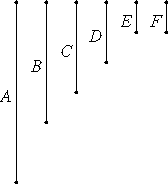# Proposition 3

To find the greatest common measure of three given numbers not relatively prime.

Let A, B, and C be the three given numbers not relatively prime.

It is required to find the greatest common measure of A, B, and C.VII.2

Take the greatest common measure, D, of the two numbers A and B. Then either D measures, or does not measure, C.

First, let D measure C.

But it measures A and B also, therefore D measures A, B, and C. Therefore D is a common measure of A, B, and C.

I say that it is also the greatest.

If D is not the greatest common measure of A, B, and C, then some number E, greater than D, measures the numbers A, B, and C.

VII.2,Cor.

Since then E measures A, B, and C, therefore it measures A and B. Therefore it also measures the greatest common measure of A and B. But the greatest common measure of A and B is D, therefore E measures D, the greater the less, which is impossible.

Therefore no number which is greater than D measures the numbers A, B, and C. Therefore D is the greatest common measure of A, B, and C.

Next, let D not measure C.

I say first that C and D are not relatively prime.

Since A, B, and C are not relatively prime, therefore some number measures them.

VII.2,Cor.

Now that which measures A, B, and C also measures A and B, and therefore measures D, the greatest common measure of A and B. But it measures C also, therefore some number measures the numbers D and C. Therefore D and C are not relatively prime.

VII.2

Take their greatest common measure E.

Then, since E measures D, and D measures A and B, therefore E also measures A and B. But it measures C also, therefore E measures A, B, and C. Therefore E is a common measure of A, B, and C.

I say next that it is also the greatest.

If E is not the greatest common measure of A, B, and C, then some number F, greater than E, measures the numbers A, B, and C.

VII.2,Cor.

Now, since F measures A, B, and C, it also measures A and B, therefore it measures the greatest common measure of A and B. But the greatest common measure of A and B is D, therefore F measures D.

VII.2,Cor.

And it measures C also, therefore F measures D and C. Therefore it also measures the greatest common measure of D and C. But the greatest common measure of D and C is E, therefore F measures E, the greater the less, which is impossible.

Therefore no number which is greater than E measures the numbers A, B, and C. Therefore E is the greatest common measure of A, B, and C.

Q.E.D.

## Guide

A common modern notation for the greatest common divisor of two numbers a and b is GCD(a, b). Also, the notation a | b is typically used to indicate that a divides b.

This proposition constructs the GCD(a, b, c) as GCD(GCD(a, b), c).

The proof that this construction works is simplified if 1 is considered to be a number. Then, two numbers are relatively prime when their GCD is 1, and Euclid’s first case in the proof is subsumed in the second.

Let d = GCD(a, b), and let e = GCD(d, c). Since e | d, d | a, and d | b, it follows that e | a and e | b, so e, in fact, is a common divisor of a, b, and c.

In order to show that e is the greatest common divisor, let f be any common divisor of a, b, and c. Then as f | a and f | b, therefore f | GCD(a, b), that is, f | d. Also, as f | d and f | c, therefore f | GCD(d, c), that is f | e. Therefore e is the greatest common divisor of a, b, and c. Q.E.D.

This is the same proposition as X.4.

This proposition is used in VII.33.